### Choose language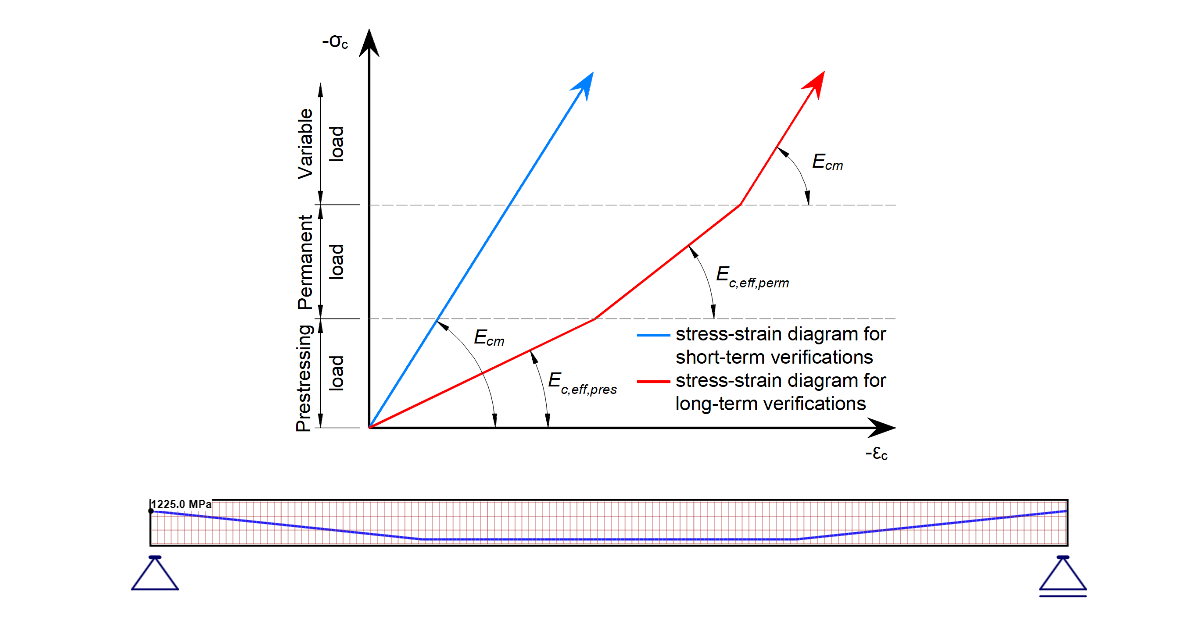# How to set self-weight for prestressed beam in Detail

$$Load cases are applied depending on the assigned Load type. Prestressing Load type Permanent Load type Variable Load type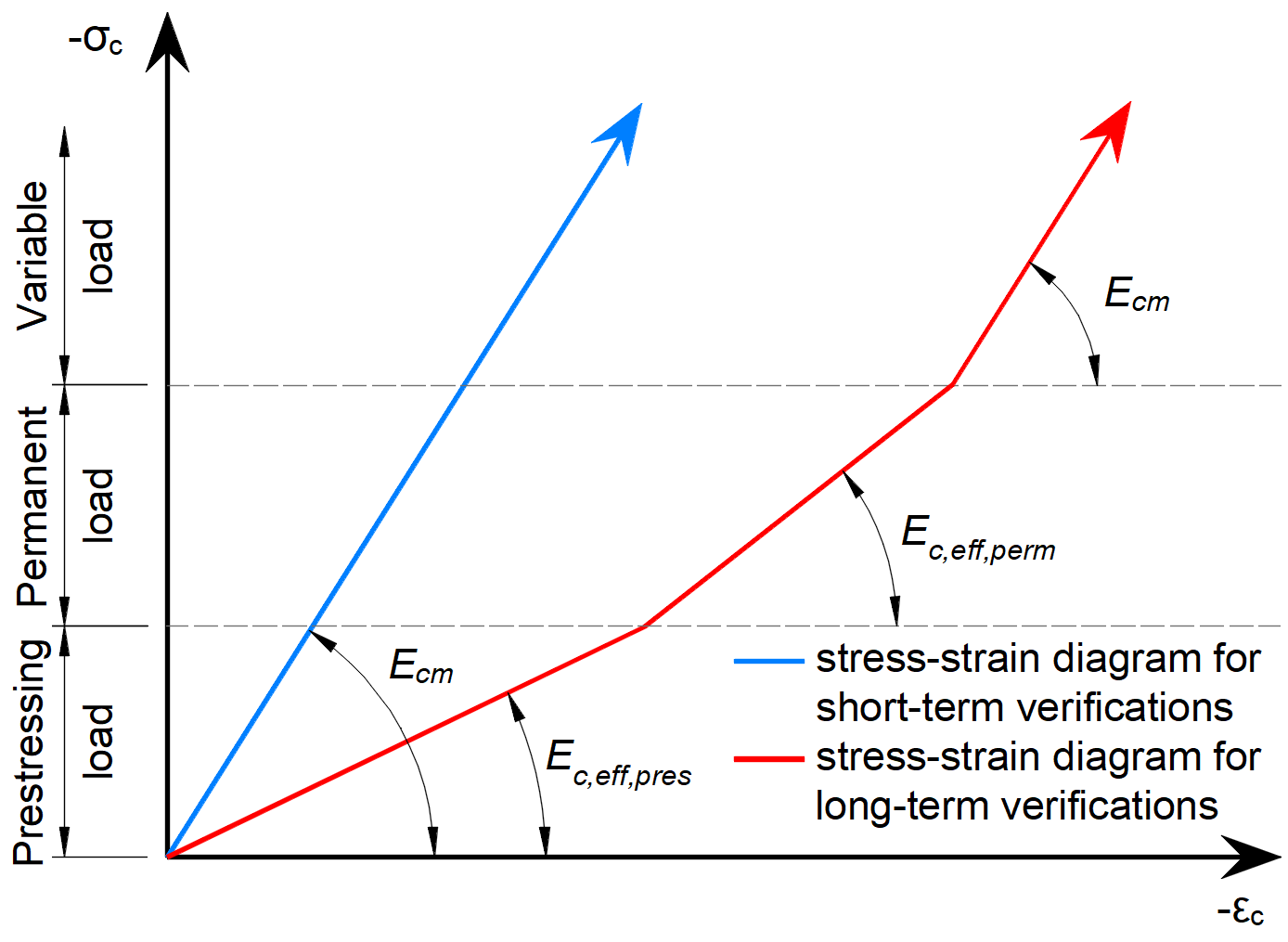The example, one span prestressed beam, is created to show the difference between setting Self-Weight as Permanent Load Case Type or as Prestressing Load Case Type. The stress in the tendon is set to 1225MPa.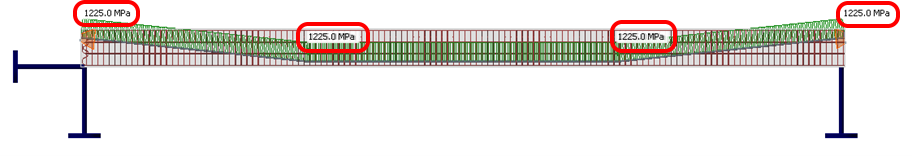Self-weight is set as Permanent Load TypeIn the first step of the calculation, the prestressing load type is applied. In this case, only the prestress itself. The stress in the cable is the specified 1225 MPa.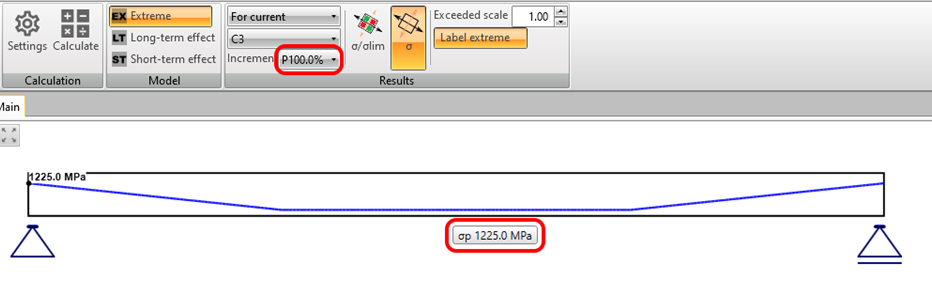The deformation in the Z direction is positive because there is only prestress applied without self-weight.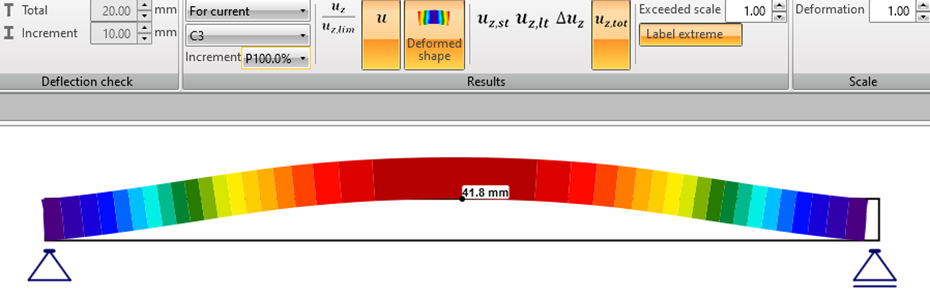In the second step of the calculation, the permanent load type is applied. In this case, the self-weight is set as the permanent load type. The deformation of the beam will be as follows: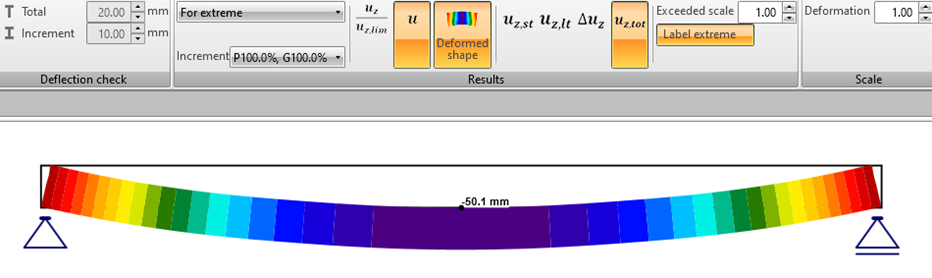The stress in the tendon is 1365 MPa. Which is 111% of the specified value. Why does this happen? Stress in the tendon is increased by the difference in the strain of the concrete fibers adjacent to the cable between the first and second steps of the calculation. How to avoid it? See below.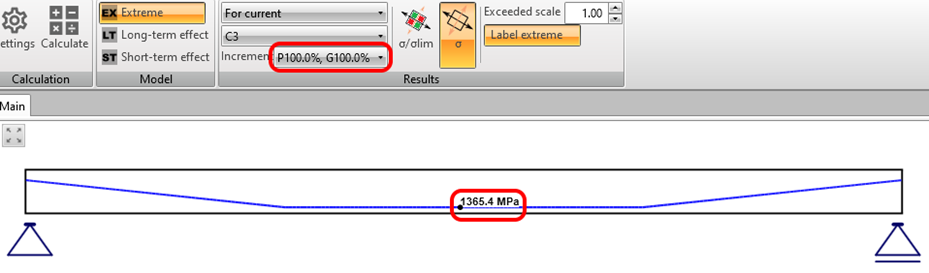Self-weight is set as Prestressing Load TypeThe same case, only the self-weight is specified as Prestressing load type.In the first step of the calculation, the prestressing load type is applied. In this case, the self-weight and prestress are set as the prestressing load type. The stress in the cable is the specified 1225 MPa.Since the self-weight is already defined in the first step of the calculation, the deformation of the beam will be the same in the first and second steps of the calculation. (No other long-term load is defined).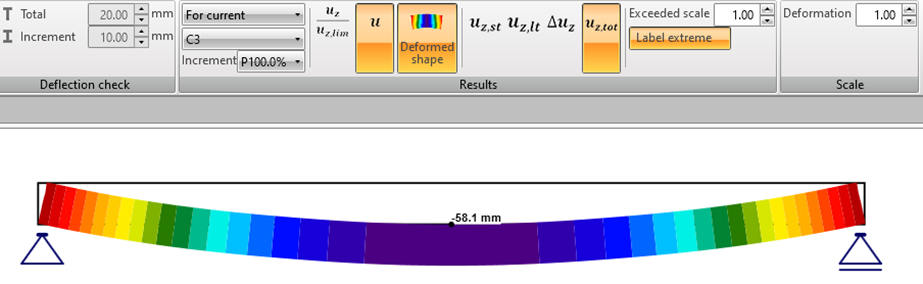ConclusionIf the self-weight is specified in the Prestressing Load type, the tendon stress in the P100%+G100% state is equal to the set value. Other long-term loads can be included in the Permanent Load type that will be applied to the structure at a later date and can therefore affect the tendon stress.It is possible to set different creep coefficients for the first and second calculation phases in the application. This means that it is possible to simulate the later time of application of other permanent loads.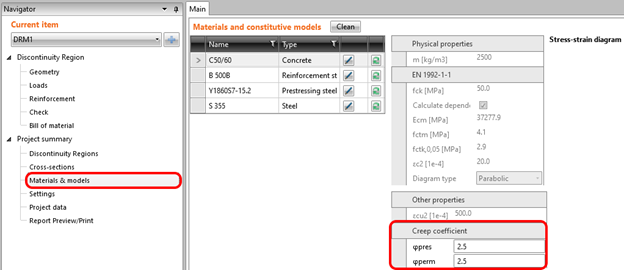Please note that both Creep coefficients used in the example had a value of 2,5.The differences in deformations in the second phase of the calculation are worth mentioning. These values indicate a possible inaccuracy.The recommended workflow is to set Self-weight as Prestressing Load Type. Related content Concrete Tutorials Prestressed beam with openings (EN) Detail EN (Eurocode) +2 Concrete Tutorials Prestressed bridge diaphragm (EN) Detail EN (Eurocode) +2 Concrete Webinars How to design a prestressed beam with openings easily? Nov 03, 2021 Detail CSFM +2 Concrete Knowledge base How to create XML file for IDEA StatiCa BIM app Beam EN (Eurocode) +3$$